# Test: Trees MCQ - 2

## 30 Questions MCQ Test RRB JE for Computer Science Engineering | Test: Trees MCQ - 2

Description
Attempt Test: Trees MCQ - 2 | 30 questions in 90 minutes | Mock test for Computer Science Engineering (CSE) preparation | Free important questions MCQ to study RRB JE for Computer Science Engineering for Computer Science Engineering (CSE) Exam | Download free PDF with solutions
QUESTION: 1

### In a binary tree, for every node the difference between the number of nodes in the left and right subtrees is at most 2. If the height of the tree is h > 0, then the minimum number of nodes in the tree is:

Solution:

Let there be n(h) nodes at height h.
In a perfect tree where every node has exactly two children, except leaves, following recurrence holds.
n(h) = 2*n(h-1) + 1
In given case, the numbers of nodes are two less, therefore
n(h) = 2*n(h-1) + 1 - 2
= 2*n(h-1) - 1 Now if try all options, only option (b) satisfies above recurrence.
Let us see option (B) n(h) = 2h - 1 + 1
So if we substitute n(h-1) = 2h-2 + 1, we should get n(h) = 2h-1 + 1
n(h) = 2*n(h-1) - 1
= 2*(2h-2 + 1) -1
= 2h-1 + 1.

QUESTION: 2

### Breadth First Search (BFS) is started on a binary tree beginning from the root vertex. There is a vertex t at a distance four from the root. If t is the n-th vertex in this BFS traversal, then the maximum possible value of n is ________ [This Question was originally a Fill-in-the-blanks Question]

Solution:

It would be node number 31 for given distance 4. For example if we consider at distance 2, below highlighted node G can be the farthest node at position 7.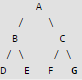QUESTION: 3

### In a binary tree, the number of internal nodes of degree 1 is 5, and the number of internal nodes of degree 2 is 10. The number of leaf nodes in the binary tree is

Solution:

In a binary tree, the number of leaf nodes is always 1 more than number of internal nodes with 2 children, refer
So, Number of Leaf Nodes = Number of Internal nodes with 2 children + 1 Number of Leaf Nodes = 10 + 1 Number of Leaf Nodes = 11

QUESTION: 4

The following three are known to be the preorder, inorder and postorder sequences of a binary tree. But it is not known which is which.
MBCAFHPYK
KAMCBYPFH
MABCKYFPH
Pick the true statement from the following.

Solution:

The approach to solve this question is to first find 2 sequences whose first and last element is same. The reason being first element in the Pre-order of any binary tree is the root and last element in the Post-order of any binary tree is the root. Looking at the sequences given,
Pre-order = KAMCBYPFH Post-order = MBCAFHPYK Left-over sequence MABCKYFPH will be in order. Since we have all the traversals identified, let's try to draw the binary tree if possible.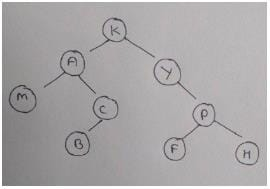I. Post order
II. Pre order
III. Inorder

QUESTION: 5

A binary tree with n > 1 nodes has n1, n2 and n3 nodes of degree one, two and three respectively. The degree of a node is defined as the number of its neighbors. n3 can be expressed as

Solution:
QUESTION: 6

A binary tree with n > 1 nodes has n1, n2 and n3 nodes of degree one, two and three respectively. The degree of a node is defined as the number of its neighbors.
Starting with the ques 5, while there remains a node v of degree two in the tree, add an edge between the two neighbors of v and then remove v from the tree. How many edges will remain at the end of the process?

Solution:
QUESTION: 7

Following function is supposed to calculate the maximum depth or height of a Binary tree -- the number of nodes along the longest path from the root node down to the farthest leaf node.

int maxDepth(struct node* node)

{

if (node==NULL)
return 0;
else
{
/* compute the depth of each subtree */
int lDepth = maxDepth(node->left);
int rDepth = maxDepth(node->right);

/* use the larger one */
if (lDepth > rDepth)
return X;
else return Y;
}

}

Q. What should be the values of X and Y so that the function works correctly?

Solution:

If a tree is not empty, height of tree is MAX(Height of Left Subtree, Height of Right Subtree) + 1 See program to Find the Maximum Depth or Height of a Tree for more details.

QUESTION: 8

What is common in three different types of traversals (Inorder, Preorder and Postorder)?

Solution:

The order of inorder traversal is LEFT ROOT RIGHT The order of preorder traversal is ROOT LEFT RIGHT The order of postorder traversal is LEFT RIGHT ROOT In all three traversals, LEFT is traversed before RIGHT

QUESTION: 9

The inorder and preorder traversal of a binary tree are d b e a f c g and a b d e c f g, respectively. The postorder traversal of the binary tree is:

Solution:

Below is the given tree.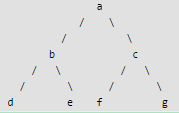QUESTION: 10

What does the following function do for a given binary tree?

int fun(struct node *root)
{
if (root == NULL)
return 0;
if (root->left == NULL && root->right == NULL)
return 0;
return 1 + fun(root->left) + fun(root->right);
}

Solution:

The function counts internal nodes. 1) If root is NULL or a leaf node, it returns 0. 2) Otherwise returns, 1 plus count of internal nodes in left subtree, plus count of internal nodes in right subtree.

QUESTION: 11

Which of the following pairs of traversals is not sufficient to build a binary tree from the given traversals?

Solution:
QUESTION: 12

Consider two binary operators '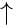' and '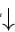' with the precedence of operatorbeing lower than that of theoperator. Operatoris right associative while operatoris left associative. Which one of the following represents the parse tree for expression (73 ­4 ­32)? (GATE CS 2011)

Solution:

Let us consider the given expression ([Tex]7 downarrow 3 uparrow 4 uparrow 3 downarrow 2[/Tex]). Since the precedence of [Tex]uparrow[/Tex] is higher, the sub-expression ([Tex]3 uparrow 4 uparrow 3[/Tex]) will be evaluated first. In this sub-expression, [Tex]4 uparrow 3[/Tex] would be evaluated first because [Tex]uparrow[/Tex] is right to left associative. So the expression is evaluated as [Tex]((7 downarrow (3 uparrow (4 uparrow 3))) downarrow 2)[/Tex]. Also, note that among the two [Tex]downarrow [/Tex] operators, first one is evaluated before the second one because the associativity of [Tex]downarrow[/Tex] is left to right.

QUESTION: 13

Which traversal of tree resembles the breadth first search of the graph

Solution:

Breadth first search visits all the neighbors first and then deepens into each neighbor one by one. The level order traversal of the tree also visits nodes on the current level and then goes to the next level.

QUESTION: 14

Which of the following tree traversal uses a queue data structure?

Solution:

Level order traversal uses a queue data structure to visit the nodes level by level.

QUESTION: 15

Which of the following cannot generate the full binary tree?

Solution:

To generate a binary tree, two traversals are necessary and one of them must be inorder. But, a full binary tree can be generated from preorder and postorder traversals. Read the algorithm here. Read Can tree be constructed from given traversals?

QUESTION: 16

Consider the following C program segment

struct CellNode
{
struct CelINode *leftchild;
int element;
struct CelINode *rightChild;
}
int Dosomething(struct CelINode *ptr)
{
int value = 0;
if (ptr != NULL)
{
if (ptr->leftChild != NULL)
value = 1 + DoSomething(ptr->leftChild);
if (ptr->rightChild != NULL)
value = max(value, 1 + DoSomething(ptr->rightChild));
}
return (value);
}

Q. The value returned by the function DoSomething when a pointer to the root of a non-empty tree is passed as argument is (GATE CS 2004)

Solution:

Explanation: DoSomething() returns max(height of left child + 1, height of left child + 1). So given that pointer to root of tree is passed to DoSomething(), it will return height of the tree. Note that this implementation follows the convention where height of a single node is 0

QUESTION: 17

Let LASTPOST, LASTIN and LASTPRE denote the last vertex visited in a postorder, inorder and preorder traversal. Respectively, of a complete binary tree. Which of the following is always true? (GATE CS 2000)

Solution:

It is given that the given tree is complete binary tree. For a complete binary tree, the last visited node will always be same for inorder and preorder traversal. None of the above is true even for a complete binary tree. The option (a) is incorrect because the last node visited in Inorder traversal is right child and last node visited in Postorder traversal is root. The option (c) is incorrect because the last node visited in Preorder traversal is right child and last node visited in Postorder traversal is root. For option (b), see the following counter example. Thanks to Hunaif Muhammed for providing the correct explanation.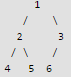Inorder traversal is 4 2 5 1 6 3 Preorder traversal is 1 2 4 5 3 6

QUESTION: 18

The array representation of a complete binary tree contains the data in sorted order. Which traversal of the tree will produce the data in sorted form?

Solution:

The level order traversal of a binary tree prints the data in the same order as it is stored in the array representation of a complete binary tree.

QUESTION: 19

Consider the following rooted tree with the vertex P labeled as root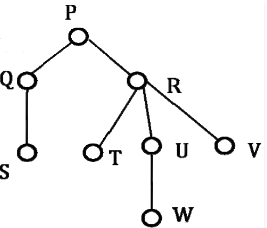Q. The order in which the nodes are visited during in-order traversal is

Solution:

Algorithm Inorder(tree) - Use of Recursion Steps:
1. Traverse the left subtree, i.e., call Inorder(left-subtree)
2. Visit the root.
3. Traverse the right subtree, i.e., call Inorder(right-subtree)
Understanding this algorithm requires the basic understanding of Recursion
Therefore, We begin in the above tree with root as the starting point, which is P.
# Step 1( for node P) :
Traverse the left subtree of node or root P.
So we have node Q on left of P.
-> Step 1( for node Q)
Traverse the left subtree of node Q.
So we have node S on left of Q.
* Step 1 (for node S)
Now again traverse the left subtree of node S which is NULL here.
* Step 2(for node S)
Visit the node S, i.e print node S as the 1st element of inorder traversal.
* Step 3(for node S) Traverse the right subtree of node S.
Which is NULL here.

Now move up in the tree to Q which is parent of S.( Recursion, function of Q called for function of S).
Hence we go back to Q.
-> Step 2( for node Q):
Visit the node Q, i.e print node Q as the 2nd element of inorder traversal.
-> Step 3 (for node Q) Traverse the right subtree of node Q.
Which is NULL here.
Now move up in the tree to P which is parent of Q.( Recursion, function of P called for function of Q).
Hence we go back to P.
# Step 2(for node P)
Visit the node P, i.e print node S as the 3rd element of inorder traversal.

# Step 3 (for node P) Traverse the right subtree of node P.
Node R is at the right of P.

Till now we have printed SQP as the inorder of the tree. Similarly other elements can be obtained by traversing the right subtree of P.

The final correct order of Inorder traversal would be SQPTRWUV.

QUESTION: 20

Consider the pseudocode given below. The function DoSomething() takes as argument a pointer to the root of an arbitrary tree represented by the leftMostChild-rightSibling representation. Each node of the tree is of type treeNode.

typedef struct treeNode* treeptr;
struct treeNode
{

treeptr leftMostChild, rightSibling;
};

int DoSomething (treeptr tree)
{
int value=0;
if (tree != NULL)
{
if (tree->leftMostChild == NULL)
value = 1;
else
value = DoSomething(tree->leftMostChild); value = value + DoSomething(tree->rightSibling);
}
return(value);
}

Q. When the pointer to the root of a tree is passed as the argument to DoSomething, the value returned by the function corresponds to the

Solution:

The function counts leaf nodes for a tree represented using leftMostChild-rightSibling representation. Below is function with comments added to demonstrate how function works. [sourcecode language="C"] int DoSomething (treeptr tree) { // If tree is empty, 0 is returned int value = 0; // IF tree is not empty if (tree != NULL) { // IF this is a leaf node, then values is initialized as 1 if (tree->leftMostChild == NULL) value = 1; // Else value is initialized as the value returned by leftmost // child which in turn calls for the other children of this node // Using last call "value = value + DoSomething(tree->rightSibling);" else value = DoSomething(tree->leftMostChild); // Add value returned by right sibling value = value + DoSomething(tree->rightSibling); } return(value); }[/sourcecode]

QUESTION: 21

Level order traversal of a rooted tree can be done by starting from the root and performing

Solution:
QUESTION: 22

Consider the label sequences obtained by the following pairs of traversals on a labeled binary tree. Which of these pairs identify a tree uniquely ?

(i) preorder and postorder
(ii) inorder and postorder
(iii) preorder and inorder
(iv) level order and postorder

Solution:

Here, we consider each and every option to check whether it is true or false. 1) Preorder and postorder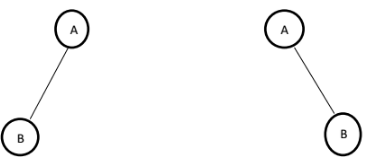For the above trees, Preorder is AB Postorder is BA It shows that preorder and postorder can’t identify a tree uniquely. 2) Inorder and postorder define a tree uniquely 3) Preorder and Inorder also define a tree uniquely 4) Levelorder and postorder can’t define a tree uniquely. For the above example, Level order is AB Postorder is BA See

QUESTION: 23

Let LASTPOST, LASTIN and LASTPRE denote the last vertex visited in a postorder, inorder and preorder traversal, respectively, of a complete binary tree. Which of the following is always true?

Solution:
QUESTION: 24

Which one of the following binary trees has its inorder and preorder traversals as BCAD  and ABCD, respectively?

Solution:

Inorder Traversal: Left -Root -Right PreOrder Traversal: Root-Left-Right

InOrder PreOrder

C.   ACBD   ABCD

Therefore, D is Correct

QUESTION: 25

The numbers 1, 2, .... n are inserted in a binary search tree in some order. In the resulting tree, the right subtree of the root contains p nodes. The first number to be inserted in the tree must be

Solution:

Binary Search Tree, is a node-based binary tree data structure which has the following properties:

• The left subtree of a node contains only nodes with keys less than the node’s key.
• The right subtree of a node contains only nodes with keys greater than the node’s key.
• The left and right subtree each must also be a binary search tree. There must be no duplicate nodes.

So let us say n=10, p=4. According to BST property the root must be 10-4=6 (considering all unique elements in BST)

And according to BST insertion, root is the first element to be inserted in a BST.

QUESTION: 26

A binary search tree contains the numbers 1, 2, 3, 4, 5, 6, 7, 8. When the tree is traversed in pre-order and the values in each node printed out, the sequence of values obtained is 5, 3, 1, 2, 4, 6, 8, 7. If the tree is traversed in post-order, the sequence obtained would be

Solution:
QUESTION: 27

If all the edge weights of an undirected graph are positive, then any subset of edges that connects all the vertices and has minimum total weight is a

Solution:

As here we want subset of edges that connects all the vertices and has minimum total weight i.e. Minimum Spanning Tree Option A - includes cycle, so may or may not connect all edges. Option B - has no relevance to this question. Option C - includes cycle, so may or may not connect all edges. Related

QUESTION: 28

When searching for the key value 60 in a binary search tree, nodes containing the key values 10, 20, 40, 50, 70 80, 90 are traversed, not necessarily in the order given. How many different orders are possible in which these key values can occur on the search path from the root to the node containing the value 60?

Solution:

There are two set of values, smaller than 60 and greater than 60. Smaller values 10, 20, 40 and 50 are visited, means they are visited in order. Similarly, 90, 80 and 70 are visited in order.
= 7!/(4!3!)
= 35

QUESTION: 29

Which of the following number of nodes can form a full binary tree?

Solution:

a full binary tree is a binary tree in which all nodes except leaves have two children.

In a Full Binary, number of leaf nodes is number of internal nodes plus 1

L = I + 1

Where L = Number of leaf nodes, I = Number of internal nodes

QUESTION: 30

Consider the following sequence of nodes for the undirected graph given below.
a b e f d g c
a b e f c g d
a d g e b c f
a d b c g e f
A Depth First Search (DFS) is started at node a. The nodes are listed in the order they are first visited. Which all of the above is (are) possible output(s)?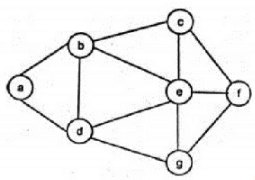Solution:

1: abef->c or g should be covered
4: adbc->e or f should be covered
2: abefcgd correctUse Code STAYHOME200 and get INR 200 additional OFF Use Coupon Code